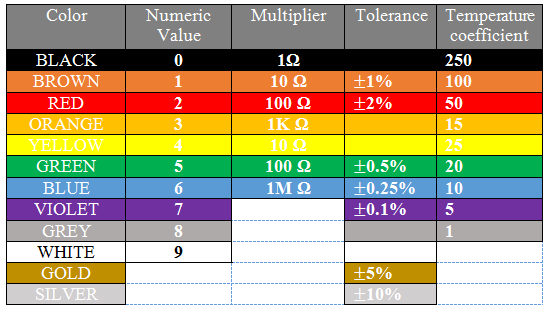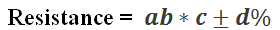# Resistor Color Code Calculator

 First Digit Second Digit Multiplier Tolerance 1 Brown 2 Red 3 Orange 4 Yellow 5 Green 6 Blue 7 Violet 8 Gray 9 White 0 Black 1 Brown 2 Red 3 Orange 4 Yellow 5 Green 6 Blue 7 Violet 8 Gray 9 White x1 Black x10 Brown x100 Red x1000 Orange x10000 Yellow x100000 Green x1000000 Blue x10M Violet x100M Gray x1G White x0.1 Gold x0.01 Silver ± 1% Brown ± 2% Red ± 3% Orange ± 4% Yellow ± 0.5% Green ± 0.25% Blue ± 0.10% Violet ± 0.05% Gray ± 5% Gold ± 10% Silver Resistance : Tolerance :

A resistor is identified by its pattern of color of bands. There are 4, 5 and 6 band resistors. To calculate the resistance of a resistor, you can select the appropriate color bands in the above resistor color code calculator.

### How to calculate the resistance?

Just have a look to the resistor color code chart below and see the calculations, how the resistance value is calculated according to this chart.### Calculating resistance for a 4-band resistorWhere,

a’ represents the 1st significant digit, which is the first band color of resistor.

b’ represents the 2nd  significant digit, which is the second band color of resistor.

c’ represents the 3rd significant digit, which is the third band color of resistor and this is the multiplier value.

d’ represents the 4th  significant digit, which is the fourth band color of resistor and this is the tolerance value.

 4-Band Name Description 1st Band a 1st significant digit 2nd  Band b 2ndsignificant digit 3rd  Band c Multiplier 4th  Band d Tolerance

Let’s take an example of a 4-band resistor with the colors given in the above image (brown, blue, red and gold).

So according to the formula the resistance will be: 16 * 100  = 1600 Ohms or 1.6K Ohms with 5% tolerance.